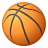# QlikView App Dev

Discussion Board for collaboration related to QlikView App Development.

cancel
Showing results for
Did you mean:Creator

## KPI Calculation with For Loop

Hi Experts

The Following is not working now , what we are trying to achieve  is Two Months Workorders

i.e This month and Previous month will fall in this month only, This is only because of Business Requirement .

Note :Extraction should start from 2014 onwards

Code :

Summary:
fd_code as [Client source],
year(floor(wo_completionDate)) as CompletionYear,
num(month(floor(wo_completionDate)),'00') as CompletionMonth,
year(floor(wo_createdDate))&'-'&num(month(floor(wo_createdDate)),'00') as CreatedYearMonth,
year(floor(wo_requestDate))&'-'&num(month(floor(wo_requestDate)),'00') as RequestYearMonth,
year(floor(wo_completionDate))&'-'&num(month(floor(wo_completionDate)),'00') as CompletionYearMonth1
from \$(vQVDDataDir)\Optimization_WO.qvd (qvd) where year(floor(wo_completionDate))='2015' and year(floor(wo_completionDate))='2014';
//and num(month(floor(wo_completionDate)),'00') >='07' and num(month(floor(wo_completionDate)),'00')<='10' ;

let vMinMonth = Min(CompletionMonth);
let vMaxMonth = Max(CompletionMonth);
let vMinYear = Min(CompletionYear);
let vMaxYear = Max(CompletionYear);

//KPI 1
for j=\$(vMinYear) to \$(vMaxYear)
for i=\$(vMinMonth) to \$(vMaxMonth)

left join(Summary)
[Client source],
count(wo_number) as [No Of Work Orders 1]
resident Summary where pri_code= 1 and  wc_code = '01'
and wo_Diff <=1
and isnull(proj_number)
and CompletionMonth >= \$(i) and CompletionMonth >= \$(i)-1
and CompletionYear >= \$(j)

// and wo_completionDate >= Addmonths(monthStart(Today()),-1) and wo_completionDate <= monthEnd(Today())
group By
[Client source]
;
next
next;

//KPI 2
for j=\$(vMinYear) to \$(vMaxYear)
for i=\$(vMinMonth) to \$(vMaxMonth)

left join(Summary)

[Client source],
count(wo_number) as [No Of Work Orders 1.1]
resident Summary  where pri_code= 1 and wc_code = '01'
and isnull(proj_number)
and CompletionMonth >= \$(i) and CompletionMonth >= \$(i)-1
and CompletionYear >= \$(j)
group by
[Client source]

;
next
next;

//KPI 3
for j=\$(vMinYear) to \$(vMaxYear)
for i=\$(vMinMonth) to \$(vMaxMonth)

left join(Summary)

[Client source],
count(wo_number) as [No Of Work Orders 2]
resident Summary  where pri_code= 2 and wc_code = '01'
and wo_Diff <=2
and isnull(proj_number)
and CompletionMonth >= \$(i) and CompletionMonth >= \$(i)-1
and CompletionYear >= \$(j)
group by

[Client source]
;
next
next;